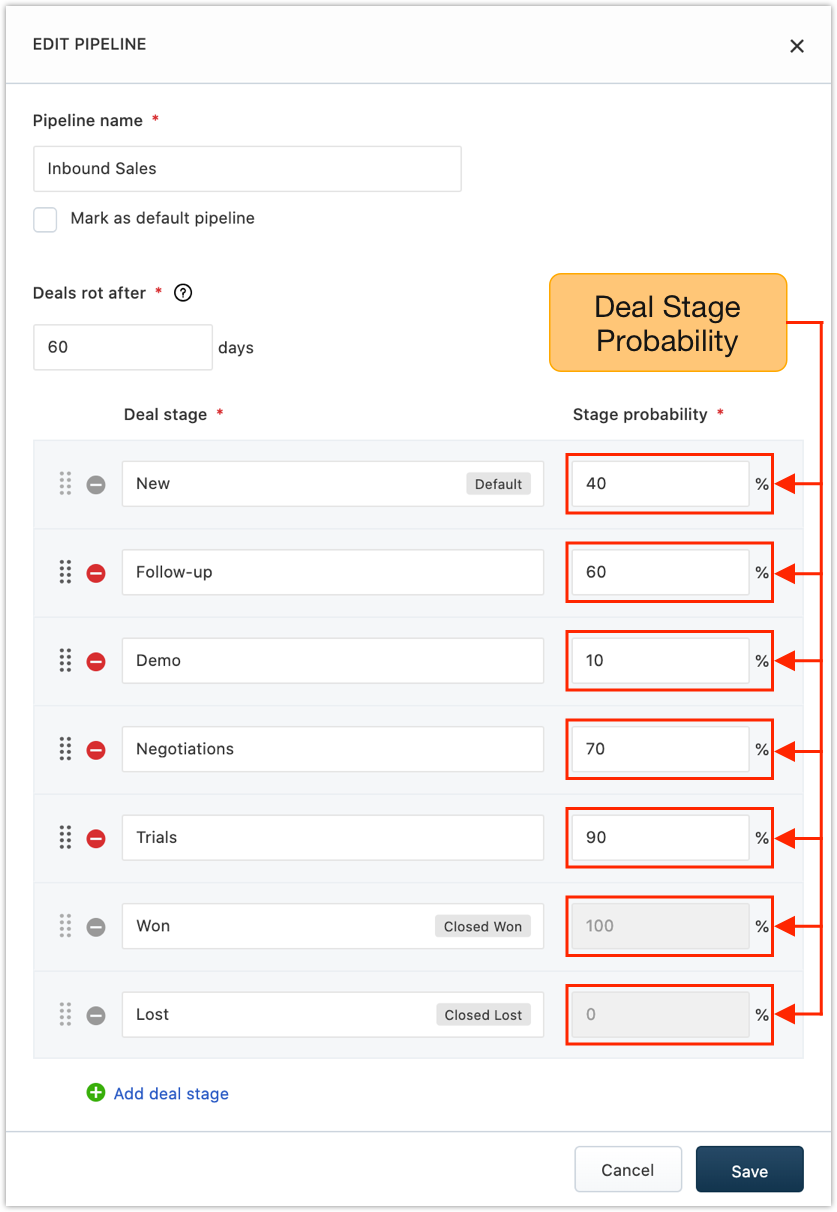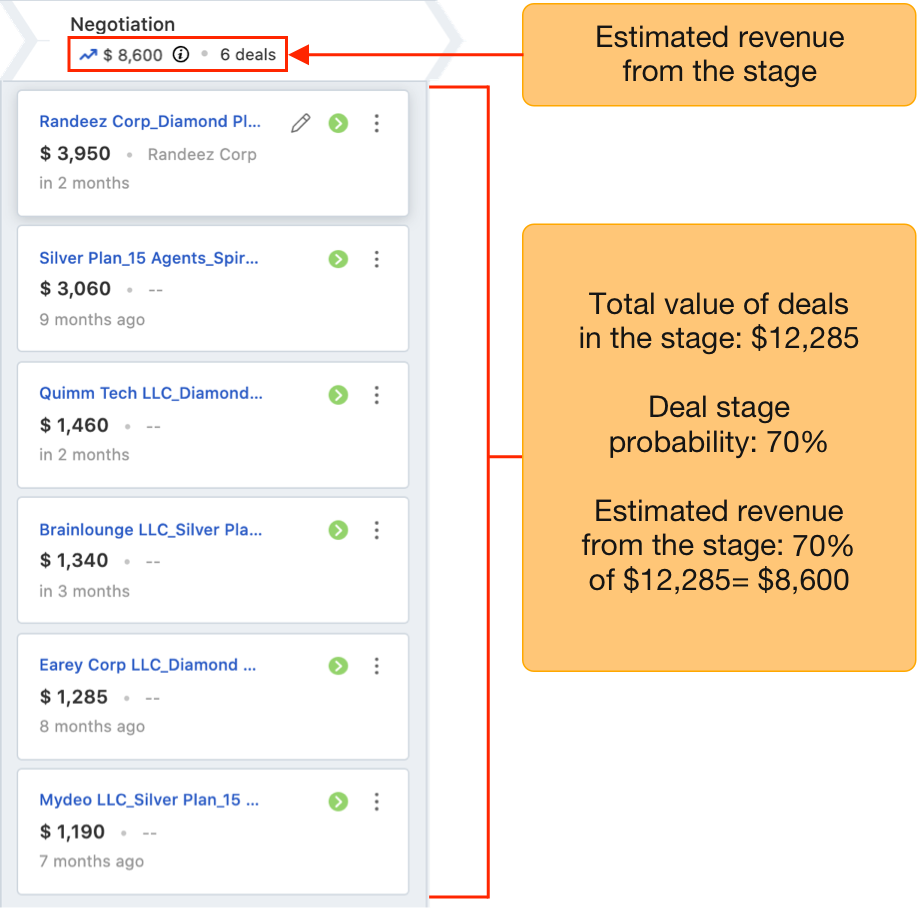Set an estimated probability against every deal stage to forecast expected sales based on the probability of deals in the pipeline.

How to set up weighted pipelines?

Here’s how:

1) Open Admin Settings and click on Pipelines under CRM Customization.

2) Click onassociated with a pipeline. This opens the pipeline and its various deal stages.

`Note: You can also assign a rotting date for the pipeline while assigning probability for the stages. Know more about it here.`

3) Assign deal stage probability for each deal stage and click. The probability is a percentage operator and operates on the total deal value of the stage. Users can change the probability of deals manually if required.`Note: When deal stage probability is edited, the new percentage will only be reflected on new deals. The percentage of existing deals will not change.`

How to forecast sales based on these probabilities?

In any given deal stage, only a fraction of deals convert successfully. By factoring in this probability for every deal stage, revenue from individual stages can be estimated realistically.

For example, let’s consider a deal stage ‘Negotiation’. At this stage of the deal cycle, the probability of winning the deal is about 70%.

Now, let us consider that you have 6 deals in this stage with each carrying values of \$3950, \$3060, \$1460, \$1340, \$1285, and \$1190 respectively.

The total deal value from this stage is \$12285.

However, the expected deal value from this stage will be calculated on the basis of the deal stage probability
(70%). Therefore, the expected revenue from this stage will be 70% of \$12285= \$8600